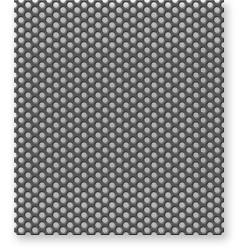# Transparency Approach (Part II)

In general, the Access Factor (AF) at any frequency is related to the Attenuation (A) at that frequency by the following formula:

AF = lO-(NlO)

### How To Use The Access Factor

In order to explain how to use the Access Factor, let us recall the definition of sound absorption coefficient, used to charac- terize the sound absorption efficiency of an acoustic treatment. We saw in Figure 12 that a 1-inch blanket of glass fiber material absorbs about 20% of the incident sound energy at a frequency of 250 Hz, about 65% at 500 Hz, about 87% at 2000 Hz and about 99% at 4000 Hz. On the other hand, a 6-inch layer absorbs about 99% of the incident energy at all frequencies.

All of these numbers assume no covering over the sound absorptive material. But when we cover the material with perforated metal, we must expect some degradation of the sound absorptive efficiency. The amount will depend on the frequency, of course, but also on the choice of the perforated metal.

The Access Factor is a measure of this degradation: it describes how much "access" the sound wave has to the underlying acoustical treatment. If the Access Factor is 1.0, there is complete access and 100% of the sound energy can get through. But if the Access Factor is 0.50, then only half the sound energy can pass through; the other half is reflected from the surface of the sheet and never reaches the acoustic treatment at all.

Therefore, in order to find the effective sound absorption efficiency of an acoustical material covered with perforated metal sheet, we simply multiply the sound absorption coefficient of the basic material at each frequency by the corresponding Access Factor for the metal sheet.

For example, suppose that we cover the 1-inch glass fiber material mentioned above, having a coefficient of 0.99 at 4000 Hz, with a perforated metal sheet having TI = 1500, corresponding to an Access Factor at 4000 Hz of 0.82. Then the effective sound ab- sorption coefficient of the combination is 0.99 x 0.82 = 0.81. The perforated covering has degraded the absorptive performance of the original material at 4000 Hz by 19 percentage points. Of course, perforated sheet with a TI of only 1500 is a poor choice for this application in the first place. The whole point of the acoustical design in the "transparency approach" is to find a sheet with as high a value of TI as possible, consistent with the other requirements of the project.

It is clear from the figures given above that if we choose a sheet with acceptable transparency at 10,000 Hz (that is, small A and high AF), then everything is much better at the lower frequencies.

The following nomogram allows you to go directly from the calculated value of TI to either the Sound Attenuation or the Access Factor, both at 10,000 Hz.

Figure 23. Nomogram for calculating the Attenuation and the Access Factor (10 kHz)

The procedure is as follows:

Enter the lower horizontal scale with the value of TI for your perforated sheet and move directly upward to intersect the lower of the two curves. Move (to the left from this intersection point until you strike the vertical scale, where you can read the attenuation at 10,000 Hz in decibels.

Alternatively, if you want the Access Factor, you can move to the right or left from the first intersection point to intersect the upper slanting line, then move upward from the second intersection point to strike the upper horizontal scale, where you can read the Access Factor at 10,000 Hz for the perforated sheet. (See Example 8, next page.)

The mathematical formulas corresponding to the curves in this nomogram are, respectively:

A(10KHz) = -22.S6log log (TI) + 0.008vn- + 13.79 dB and
AF = 10-(N10).

The first formula is valid for values of TI up to 50,000, but only for a frequency of 10,000 Hz; the second formula is generally valid for any frequency for which the value of A is known.

### Example 8

Suppose you have a perforated metal sheet with TI = 4000, used as a covering for a sound absorptive glass fiber blanket. What is the effect of the covering? Enter the lower horizontal scale of Fig. 23 at TI = 4000, move upward to strike the lower curve, then move left to find an attenuation at 10,000Hz of 1.8 dB. Next, move to the right from the first intersection point to intersect the upper slant line, then upward from this point to the horizontal scale to find an Access Factor of 0.66. With this sheet covering an absorptive material, you will realize only 66% of the intrinsic absorption performance of the glass fiber material at 10,000 Hz.

NOTE: See Appendix C for an important technical qualification to the use of the Access Factor, as prescribed above.

NOTE: Full-sized, clean versions of Figs. 21, 22 and 23 are included in Appendix D at the back of this booklet, to be copied and used as worksheets.

Table 1 presents calculated values of the TI, the Attenuation (A) and the Access Factor (AF) at 10,000 Hz, for a group of the most commonly manufactured perforated metals.